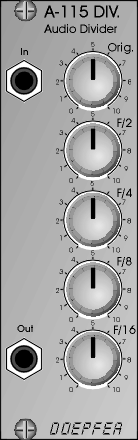﻿ A-115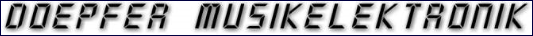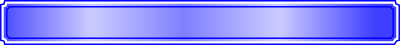Analogue  Modular  System  A-100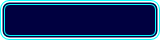WidthHPDepth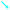mmCurrentmA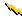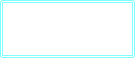Owners ManualAudio Divider

The Audio Divider is a four way frequency divider. The frequency of a signal at the input is halved (1st sub-octave), quartered (2nd sub-octave) and so on. In this way, the Divider produces four sub-octaves (F/2, F/4, F/8 and F/16). At the output, the A-115 produces a summed mix of the original and the four sub-octaves.

There are attenuators to control the amount (level) of the original signal and each of the sub-octaves. All sub-octave outputs are true square waves. At the output there can be four square waves plus the original signal forming a composite “staircase” waveform.

8

40

10HP

20

10HP

A-115